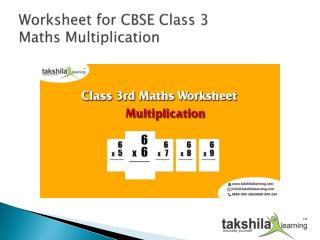DownloadDownload PresentationMental Maths for kids topic is Multiplication | Worksheet for Class 3 Maths

# Mental Maths for kids topic is Multiplication | Worksheet for Class 3 Maths

Download Presentation## Mental Maths for kids topic is Multiplication | Worksheet for Class 3 Maths

- - - - - - - - - - - - - - - - - - - - - - - - - - - E N D - - - - - - - - - - - - - - - - - - - - - - - - - - -
##### Presentation Transcript

1. Worksheet for CBSE Class 3 Maths Multiplication

2. Worksheet for CBSE Class 3 Maths Multiplication Multiplication Fill in the blanks : • Multiplication is repeated……………………… • Any number multiplied by 0, the product is always……………………… • The product of any number and 1 is number………………………… • To multiply by multiples of 10, put as many …………………….. at the end of the product as there are in the multiplier. • 2 + 2 + 2 + 2 =   ………….. x …………… .

3. Worksheet for CBSE Class 3 Maths Multiplication • …………. x 365 = 0 • 2 dozen apples = ………….. apples. • 4 chairs have………….. legs. • 365 x 1 x 1 x 1 = ………… • 34 x 1 x 2 = ……….. • 560 x 10 = ………….. • 3 x 8 = 20 + ………… • 12 pairs = ………… dozen. • 8 times 7 is …………. • ……….. x 9 = 63. • 6 x 3 = 18, 3 x 6 = ……………. • The answer of the multiplication is called the ………………….

4. Worksheet for CBSE Class 3 Maths Multiplication How many legs do? • 9 lions have ………………… • 6 spiders have …………………. • 5 snakes have …………………… • 8 boys have ……………………. • 10 dogs have ……………………… • 7 birds have ………………………

5. Worksheet for CBSE Class 3 Maths Multiplication Mutiply : • (1)  5    6    9                                             (3)     3     4     8                                  (2)      6    5    8         x    8       x  1    2      x   1   3 (4)   3    9    4                                             6)     4    9     7                                     (5)        3     4     0     x  2    4     x    1    8    x    2    9

6. Worksheet for CBSE Class 3 Maths Multiplication Clear your concept of Multiplication with animated learning module, and practice with lots of worksheets. Click Class 3rd Maths for more details. Find the product: • 87 x   10    = ………………….. • 52 x   100  =  ………………….. • 348 x  10    =  ………………….. • 675 x  10   =  …………………. • 30 x   100   =  …………………. • 8 x  1000   =  …………………. Find the product : • 28 x   ………..    =   280 • 16 x   ………..    =   1600 • ……….. x 25        =   250 • ………. x  68       =    6800 • ………… x  15      =    1500 • ………… x  8600 =    0

7. Worksheet for CBSE Class 3 Maths Multiplication Find the product : • 32 x   40   =   ………………….. • 82 x    90   =   ………………… • 368 x   20   =   ………………… • 333 x   30   =   …………………. • 56 x   80   =   …………………. • 16 x   300 =   …………………. Solve the following word problems: • Meera eats 3 chapattis every day. In 1 week, how many chapattis has she eaten? • The cost of 1 kg of milk is Rs. 36. What will be the cost of 87 kg of Milk? • One day has 24 hours. How many Hours are there in 15 days?

8. Worksheet for CBSE Class 3 Maths Multiplication • Download CBSE Class 3 Maths Multiplication Worksheet Find out more worksheets of Class 3rd Maths, Science, and English. Register with Takshilalearnig and see the difference in your kid’s performance. We provide Animated learning content, games, worksheets, olympiad practice papers and much more. Visit our website for more details www.takshilalearning.com • Click below and Subscribing to our social channels • Follow us on Blogarama • Worksheets for Class 3 : Call us: 8800999280/8800999284